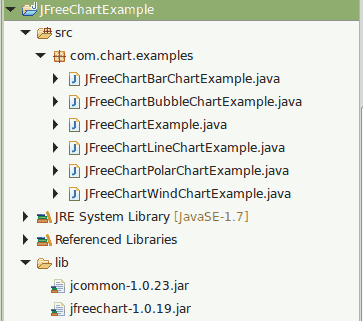# Create Bar Chart Using JFreeChart

Create Bar Chart Using JFreeChart explains about creating a simple bar chart using JFreechart API

A bar chart or bar graph is a chart with rectangular bars with lengths proportional to the values that they represent. The bars can be plotted vertically or horizontally. A vertical bar chart is sometimes called a column bar chart

Reference -> http://en.wikipedia.org/wiki/Bar_chart

How To Create Bart Chart Using JFreeChart library?

JFreeChart is a free and open source java chart library used for creating professional quality charts. JFreeChart is purely written in java language, we can very easily incorporate JFreeChart in our java standalone and web applications.

Referenece - > http://www.jfree.org/jfreechart/

Here I am showing an example about How to create  a simple Bar Chart using JFreeChart.

Note

1) If you need pie chart example, You can follow Create Pie Chart Using JFreeChart

2) If you need line chart example, You can follow Create Line Chart Using JFreeChart

3) If you need bar chart example, You can follow Create Bar Chart Using JFreeChart

4) If you need polar chart example, You can follow Create Polar Chart Using JFreeChart

5) If you need bubble chart example, You can follow Create Bubble Chart Using JFreeChart

6) If you need wind chart example, You can follow Create Wind Chart Using JFreeChart

###### Required Libraries

Following jar must be in classpath

1. jfreechart-1.0.13.jar
2. jcommon-1.0.16.jar

We can create following professional quality charts by using jfreechart.

• Single valued Charts such as compass, speedometer, thermometer
• Line Chart
• Pie Chart
• Bar Chart
• Bubble Chart
• Wind Chart
• Polar Chart

## Project Structure1 Responses to "Create Bar Chart Using JFreeChart"
1. Nazeer 2016-06-08 06:03:40.0